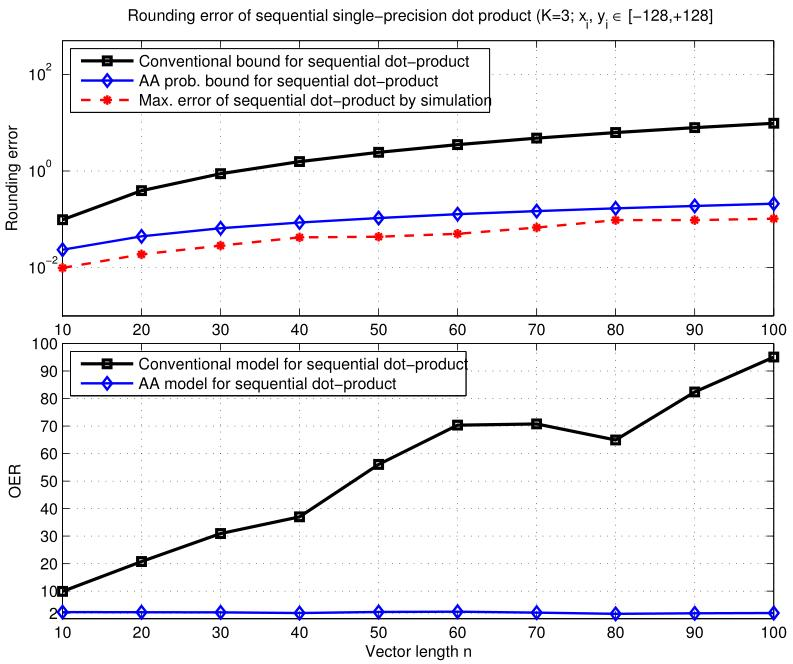Signal Processing and Speech Communication Laboratory

## Efficient Floating-Point Implementation of Speech Processing Algorithms on Reconfigurable Hardware

Status
Finished
Student
Thang Huynh Viet
Mentor
Gernot Kubin
Research AreasThis doctoral thesis aims at optimising the ﬂoating-point implementations of signal processing algorithms on reconﬁgurable hardware with respect to accuracy, hardware resource and execution time. It is known that reduced precision in ﬂoating-point arithmetic operations on reconﬁgurable hardware directly translates into increased parallelism and peak performance. As a result, efficient implementations can be obtained by choosing the minimal acceptable precision for ﬂoating-point operations. Furthermore, custom-precision ﬂoating-point operations allow for trading accuracy with parallelism and performance. We use Affine Arithmetic (AA) for modeling the rounding errors of ﬂoating-point computations. The derived rounding error bound by the AA-based error model is then used to determine the smallest mantissa bit width of custom-precision ﬂoating-point number formats needed for guaranteeing the desired accuracy of ﬂoating-point applications.

In this work, we implement the ﬁrst Matlab-based framework for performing rounding error analysis and numerical range evaluation of arbitrary ﬂoating-point algorithms using the AA-based error model. Our framework enables users to best reuse their own existing Matlab code to eﬀectively conduct rounding error analysis tasks and run bit-true custom-precision computations of ﬂoating-point algorithms in Matlab for veriﬁcation.

We apply the AA-based error analysis technique and our Matlab-based framework to the ﬂoating-point rounding error evaluation and optimal uniform bit width allocation of two signal and speech processing applications: i) the ﬂoating-point dot-product and ii) the iterative Levinson-Durbin algorithm for linear prediction and autoregressive modeling. For the ﬂoating-point dot-product, it is shown that the AA-based error model can provide tighter rounding error bounds compared to existing error analysis techniques. This corresponds to the overestimation of up to 2 mantissa bits compared to those estimated by running extensive simulations. For the iterative Levinson-Durbin algorithm, the AA-based error analysis technique can model accurately the rounding errors of the coeﬃcients when using a restricted range for the input parameters. When using a general range for the input parameters, the AA-based error analysis technique can give a qualitative estimate for the error bound of the coeﬃcients.